# MPEG Motion Estimation

Alex Egg,

This is the 3rd part in a series about multimedia compression. See the second part on JPEG compression here: http://www.eggie5.com/47-jpeg-compression

## Motivation

A simple uncompressed video file will contain an array of 2D buffers containing pixel data for each frame. So it’s a 3D (2 spatial dimensions and 1 temporal) array of bytes. Each pixel takes 3 bytes to store - one byte each for the three primary colors (red, green and blue).

1080p @ 60 Hz = 1920x1080x60x3 => ~370 MB/sec of raw data.

This is next to impossible to deal with. A 50GB Blu-ray disk will only hold ~2 mins. You can’t move it anywhere fast. Even SSDs have trouble dumping this straight from RAM to Disk1.

So yeah. We need compression.

As we established, raw video is too large to store and transmit practically. Just like still image JPEG compression, video compression exploits spatial redundancies. However, for the video medium, removal of spatial redundancy is not enough to achieve the necessary level of compression for transmission and storage of high-def. video. In addition to spatial redundancy, temporal redundancy exploitation is central to most video compression techniques. Temporal redundancies are redundancies not in the domain of space or a single frame, but redundancies over-time. For example, video is often taken at 30 frames/second and even while a video sequence may seem fast-motion many redundancies can be seen between one from to the next. For example, at 30 frames/second, frame #1 is captured at time 0 and frame #2 is captured at time 33 milliseconds and frame #3 is captured at 66 milliseconds. Typically, the difference between frame 3 and 1 is small and the difference is even smaller between from 2 and 1 which are only 33 ms apart. If a compression method takes advantage of these temporal redundancies, it can achieve a high-degree of compression.

To exploit temporal-redundancy in video, a technique called motion-estimation is employed. Though motion estimation, we try to track the x,y pixel shift of a block form one form to the next. This shift can be quantified as a vector. Then using the reference frame + the shift motion vectors we can recreate the frame after the reference from w/o explicitly storing its data. We recreate it using the data from the reference and the block shifts. The technique of matching the blocks in the current frame w/ the reference from is called block matching and the creation of the motion vectors is called motion estimation. With the combination of these 2 techniques we can recreate frames from a source (reference) and thus achieve a degree of compression. We will explore these techniques in this lab.

#Procedure

To conduct this research I had to create two block-search algorithm implementations: exhaustive search and a faster method of choice: Three Step Search (TSS). Exhaustive search is the naive approach and comes w/ the highest computation overhead, however, it is easy to implement. TSS is a more sophisticated algorithm w/ a lower computational complexity however, it is more difficult to code.

##Exhaustive Search

In Exhaustive search the the block of the current frame is matched w/ a block in the reference frame. A search window is setup in the reference frame around the perimeter of the block. The search then iterates every possible location within the search window recording error between the sample and the block from the current frame. The error can be quantified by various means, for example: MSE, MAD or SAD. In previous labs in this class we used MSE, however, MSE will incur too large an overhead in a real-world implementation so we defer to MAD, or mean absolute difference which will give us a similar metric as MSE. We take the error (MSE) for each block in the search window and the block w/ the smallest error can be considered the best match for the block in the current frame. We then measure the displacement difference and save this as the motion vector. We then move the next block in the current frame and repeat the process. An example of the search process can be seen the figure 1 below:Figure 1: Block Matching a MB of 16 pixels and a search window p of size 7 pixels.

The ES algorithm calculates the cost function at each possible location in the search window. As a result of which it finds the best possible match and gives the highest PSNR amongst any block matching algorithm. Fast block matching algorithms try to achieve the same PSNR doing as little computation as possible. The obvious disadvantage to ES is that the larger the search window gets the more computations it requires.

##Three Step Search (TSS)

Like most algorithms in live there is a naive approach and usually more sophisticated approaches. If we just add a heuristic to our algorithm we can achieve a faster runtime and lower computational complexity. There are various algorithms that improve upon exhaustive search, but the one I chose to implement is TSS. This is one of the earliest attempts at fast block matching algorithms and dates back to mid 1980s. The general idea is represented in Figure 2. It starts with the search location at the center and sets the ‘step size’ S = 4, for a usual search parameter value of 7. It then searches at eight locations +/- S pixels around location (0,0). From these nine locations searched so far it picks the one giving least cost and makes it the new search origin. It then sets the new step size S = S/2, and repeats similar search for two more iterations until S = 1. At that point it finds the location with the least cost function and the macro block at that location is the best match. The calculated motion vector is then saved for transmission.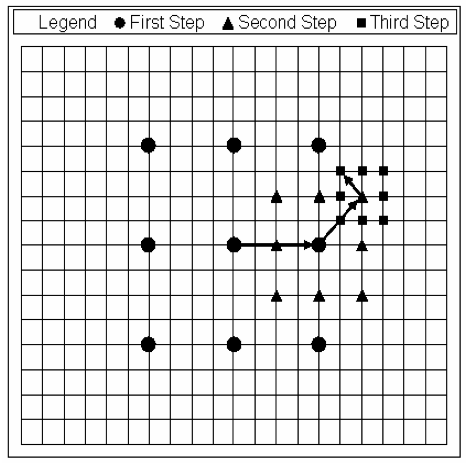Figure 2: Three Step Search procedure. The motion vector is (5, -3).

After I implemented these algorithms, I ran them against 5 frames of the walk_qcif.avi video provided for the lab. The results are documented in the next section.

#Results

In summary, the ES method provided the best performance in terms of PSNR however, it is slow computationally. TSS was orders of magnitude faster than ES and at only a small loss of PSNR.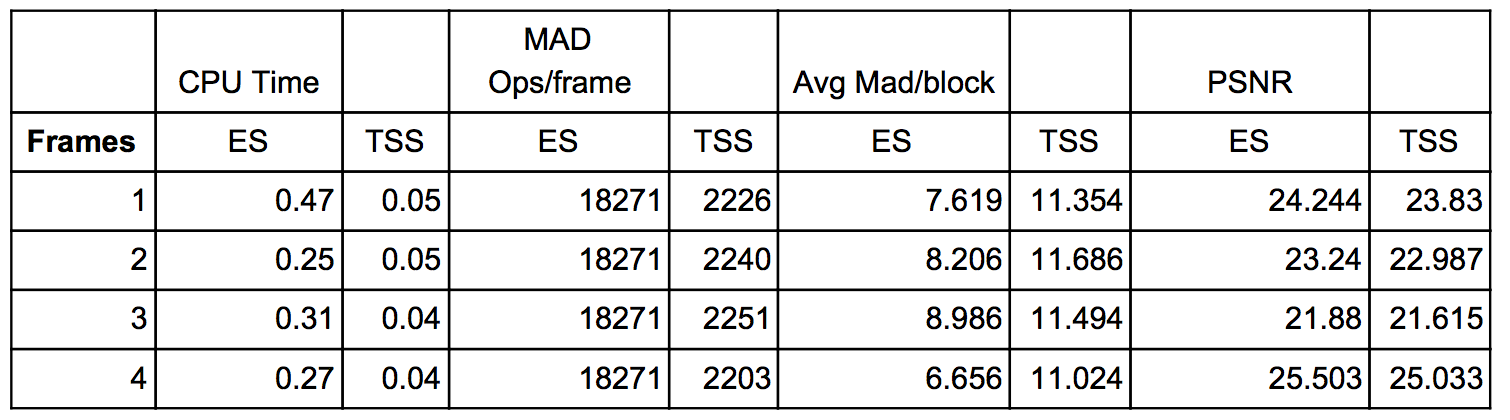Table 1: Statistics Across all frames

As we can see from Table 1, the CPU time involved for TSS is almost 10 times smaller. Also, the CPU time for TSS is stable, while ES CPU time does not seem to converge on a value, but rather oscillates. This behavior can be understood by an explanation of the algorithms. TSS uses a heuristics to track down the smallest MAD difference which allows it to work in constant time. However, ES uses no heuristic, and just searches blindly, thus producing random search times.

We can also, see from table 1 that the number of MAD operations per frame with TSS around 8 times smaller than ES. Another interesting note, is that w/ ES is constant, while TSS has variation. This has a similar explanation as the CPU explanation above. ES performs the same exact search routine every time, and thus check the same amount of blocks every time. While, TSS uses a heuristic to more intelligently search blocks.

Also another interesting metric is in the PSNR columns. This is ES only redeeming quality. While it bring poor performance in the other metrics it does give a higher PSNR. However, it is not that much larger than TSS, which means TSS is a far superior algorithm, with almost no quality loss over ES.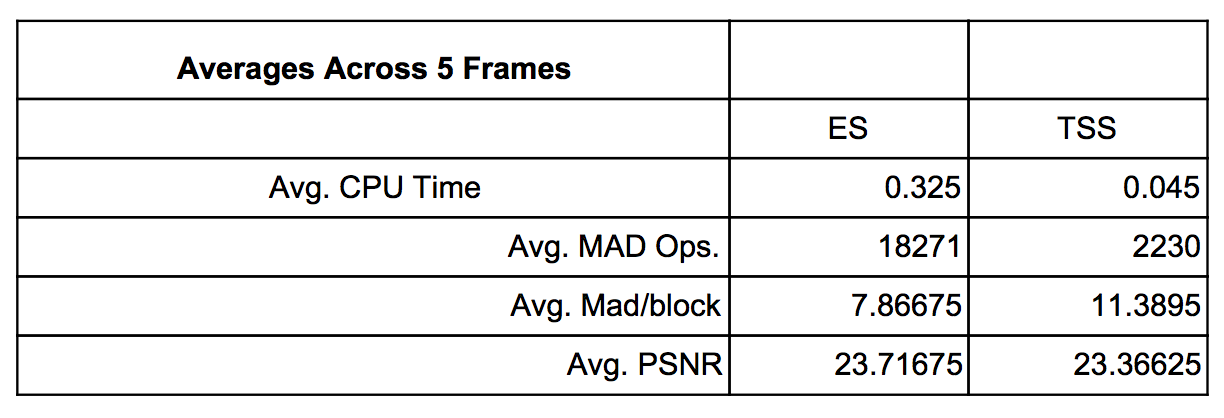Table 2: Statistics averaged across all frames

Table 2 summaries the results from the above analysis. CPU time is about 7 times faster w/ TSS with an equal amount of PSNR error.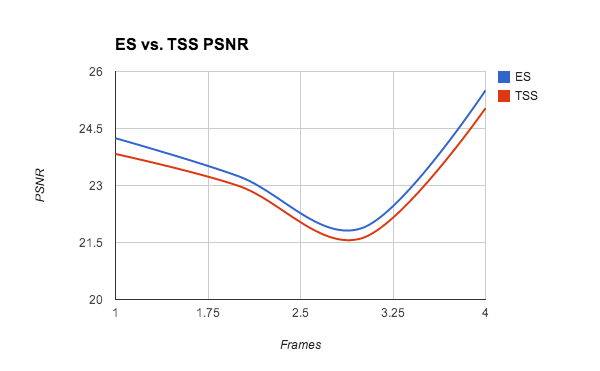Figure 3: A comparison of PSNR for ES vs. TSS.Figure 4: TSS algorithm vs the time-varying ES algorithm.

List of Figures: Motion Vectors, Reconstructed & Error Images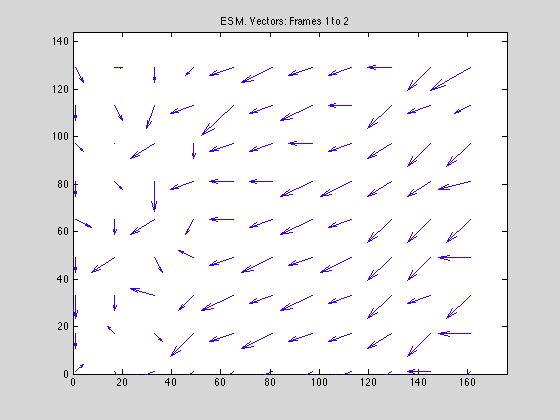Figure 5Figure 6Figure 7Figure 8Figure 7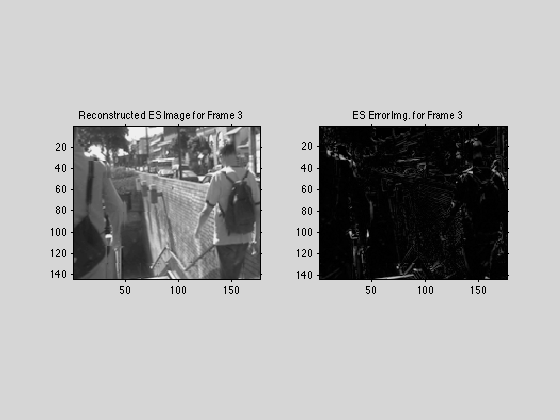Figure 8Figure 9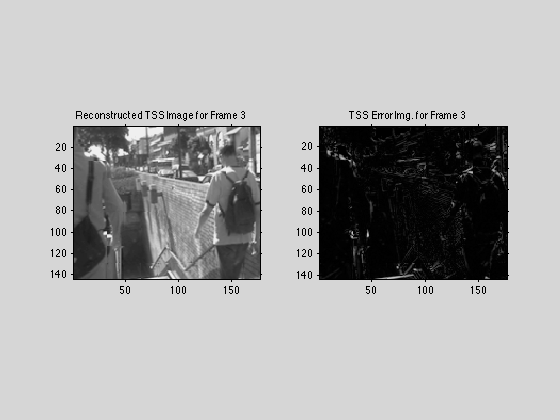Figure 10Figure 11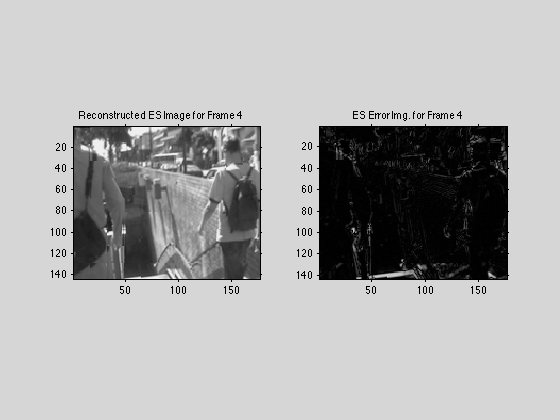Figure 12Figure 13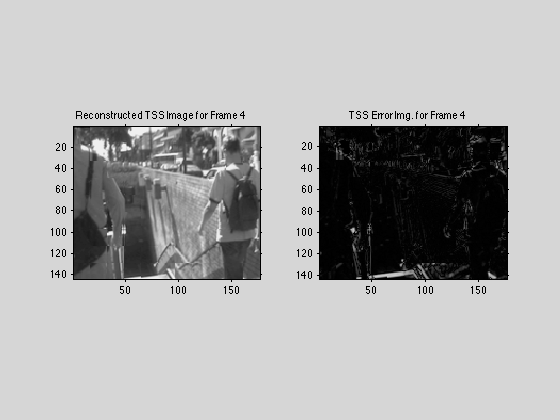Figure 14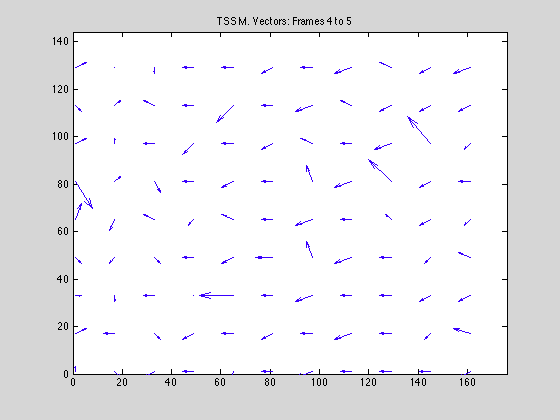Figure 15Figure 16Figure 17

# Conclusion

Ultimately, throughout this project we have succeeded in learning to read and display video in Matlab, break video into frames, and learn Motion Estimation techniques for reducing the temporal redundancy in video sequences. I believe the main goal of this project was to teach us the basics of video/image processing, while gearing us toward more compression programming.

We introduced to the practice of motion estimation in order to predict the the next frame. This uses redundancy to find to the best match and determine the motion vectors from one frame to another. We displayed this using quiver from Matlab. By finding the best match, the next frame can be predicted based upon the entire motion of the frame. This method only takes into account motion in a 2 dimensional way. If a person were to turn to the side, it would have a difficult time tracking this motion. The image is broken down into MacroBlocks and then each one is evaluated in this way. We used two different methods to find the best match, exhaustive and conjugate. Just by running the program it is easy to see that exhaustive takes much more time than the conjugate.

The compression of videos is an important part of society and this is just the first step of many to accomplish this. Compression is necessary but it must also take into consideration noise and how the end user will view the image. The next step is to use this method and add in more compression aspects such as DCT and quantization for each frame. Each frame must then be encoded separately and sent to the decoder. This means that headers become necessary to tell the decoder frame number and other useful information. Possibly the next step will include audio compression as well however this may be a separate class altogether.

Permalink: mpeg-motion-estimation

Tags:

Last edited by Alex Egg, 2016-11-05 03:16:44